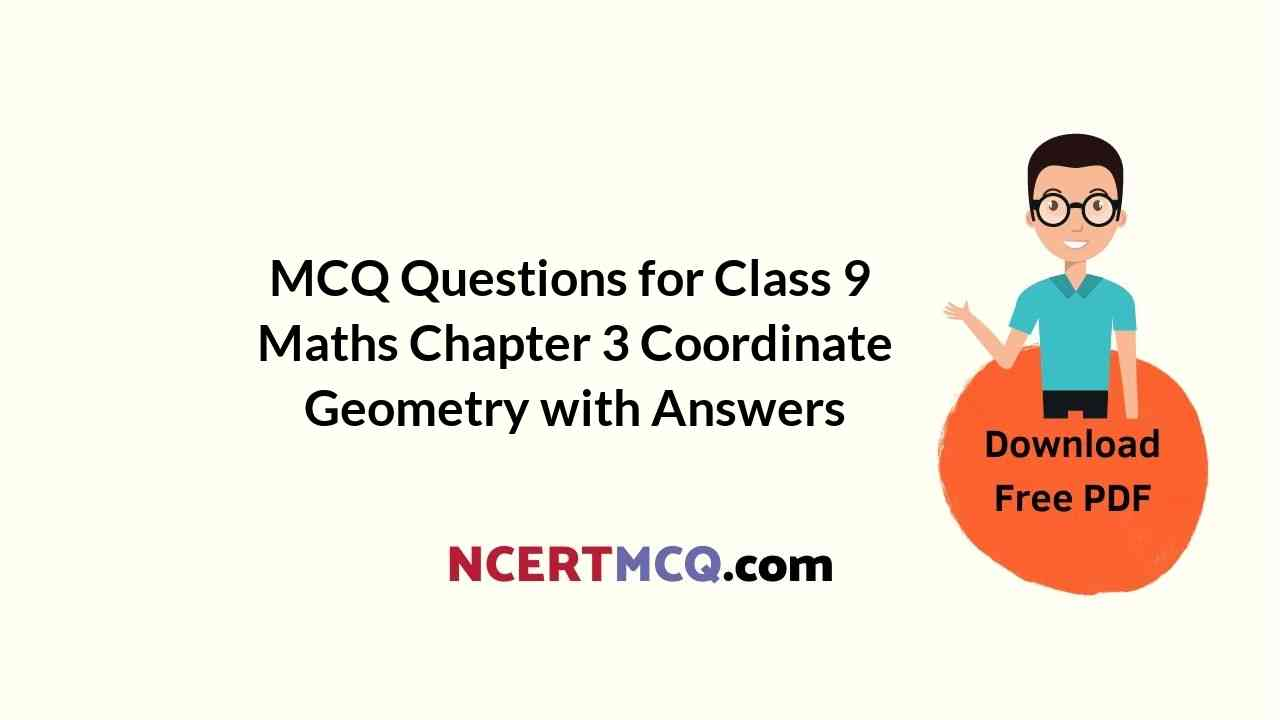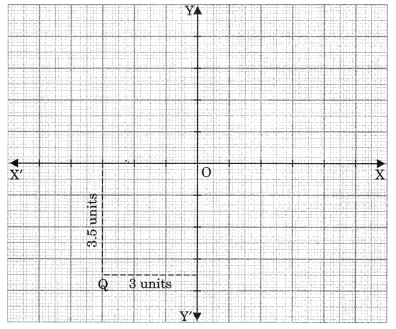Check the below Online Education NCERT MCQ Questions for Class 9 Maths Chapter 3 Coordinate Geometry with Answers Pdf free download. MCQ Questions for Class 9 Maths with Answers were prepared based on the latest exam pattern. We have provided Coordinate Geometry Class 10 Maths MCQs Questions with Answers to help students understand the concept very well. https://ncertmcq.com/mcq-questions-for-class-9-maths-with-answers/

Students can also refer to NCERT Solutions for Class 9 Maths Chapter 3 Coordinate Geometry for better exam preparation and score more marks.

## Online Education for Coordinate Geometry Class 9 MCQs Questions with Answers

Coordinate Geometry Class 9 MCQ Question 1.
Abscissa of a point is positive in

Class 9 Maths Chapter 3 MCQ Question 2.
The points (-5, 2) and (2, -5) lie in the
(b) II and III quadrant respectively.
(c) II and IV quadrant respectively.
(d) I and IV quadrant respectively.

Coordinate Geometry MCQ Class 9 Question 3.
If (x + 2, 4) = (5, y – 2), then coordinates (x, y) are
(a) (7, 12)
(b) (6, 3)
(c) (3, 6)
(d) (2, 1)

Class 9 Coordinate Geometry MCQ Question 4.
Mirror image of the point (9, -8) in y-axis is
(a) (-9, -8)
(b) (9, 8)
(c) (-9, 8)
(d) (-8, 9)

Ch 3 Maths Class 9 MCQ Question 5.
The coordinates of the point which lies on y-axis at a distance of 4 units in negative direction of y-axis is
(a) (5, 4)
(b) (4, 0)
(c) (0, -4)
(d) (-4, 0)

Class 9 Maths Ch 3 MCQ Question 6.
If the points A(2, 0), B(-6, 0) and C(3, a – 3) lie on the x-axis, then the value of a is
(a) 0
(b) 2
(c) 3
(d) -6

MCQ Of Coordinate Geometry Class 9 Question 7.
Which of the following points lies on the negative side of x axis?
(a) (-4, 0)
(b) (3, 2)
(c) (0, -4)
(d) (5, -7)

MCQ On Coordinate Geometry For Class 9 With Answers Question 8.
The Coordinates of point Q are(a) (3, 3.5)
(b) (3.5, 3)
(c) (-3, 3.5)
(d) (-3, -3.5)

Chapter 3 Maths Class 9 MCQ Question 9.
The point M lies in the IV quadrant. The coordinates of point M are
(a) (a, b)
(b) (-a, b)
(c) (a, -b)
(d) (-a, -b)

Class 9 Maths Coordinate Geometry MCQ Question 10.
Write the name of the quadrant in which the point (-3, -5) lies.

Class 9 Maths Chapter 3 MCQ With Answers Question 11.
The number of parts the coordinates axes divide the plane is
(a) Two parts
(b) Four parts
(c) Six parts
(d) Eight parts

Class 9th Maths Chapter 3 MCQ Question 12.
Point (0, 4) lies
(b) on x-axis
(c) on y-axis

MCQ Questions For Class 9 Maths Coordinate Geometry Question 13.
The mirror image of the point (-3, -4) in x-axis is
(a) (-4, -3)
(b) (3, -4)
(c) (3, 4)
(d) (-3, 4)

Class 9 Chapter 3 Maths MCQ Question 14.
In which quadrant does the point (-1, 2) lies?

MCQ Questions For Class 9 Maths Ncert Chapter 3 Question 15.
Abscissa of a point is negative in

Question 16.
Abscissa of all the points on y-axis is
(a) 1
(b) any number
(c) 0
(d) -1

Question 17.
Which is the example of geometrical line?
(a) Blackboard
(b) Sheet of paper
(c) Meeting place of two walls
(d) Tips of sharp pencil.